Question

11. What are the possible combination outcomes when you toss a fair coin three times? (6.25...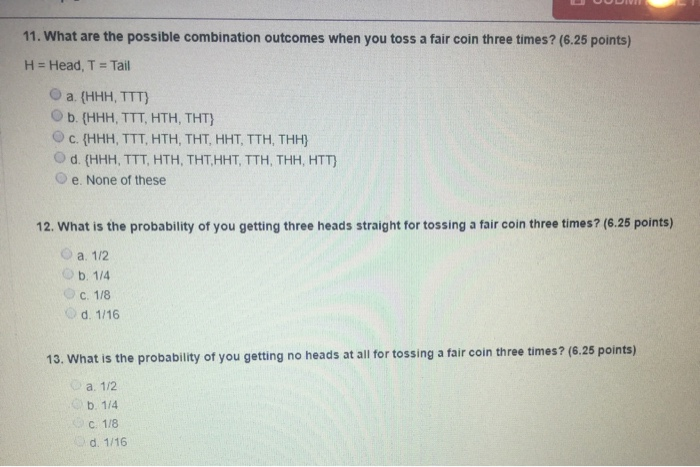11. What are the possible combination outcomes when you toss a fair coin three times? (6.25 points) H = Head, T = Tail a {HHH, TTT) Ob. (HHH, TTT, HTH, THT) c. {HHH, TTT, HTH, THT, HHT, TTH, THH) d. (HHH, TTT, HTH, THT, HHT, TTH, THH, HTT} e. None of these 12. What is the probability of you getting three heads straight for tossing a fair coin three times? (6.25 points) a. 1/2 OD. 1/4 C. 118 d. 1/16 13. What is the probability of you getting no heads at all for tossing a fair coin three times? (6.25 points) . a. 112 D. 114 C 1/8 d. 1/16

11) What are the possible combination outcomes when you toss a fair coin three times?

The correct answer is Option (d):

There are 8 possible outcomes when we toss a fair coin three times.

12) What is the probability of you getting three heads straight for tossing a fair coin three times?

The correct answer is Option (c):

The sample space for tossing a fair coin three times is,

Number of possible outcomes in sample space

Let A be the event of getting three heads.

Number of outcomes with three heads

We know that,

Probability = Number of favourable outcomes / Total number of outcomes

The probability of getting three heads straight for tossing a fair coin three times is,

13) What is the probability of you getting no heads at all for tossing a fair coin three times?

The correct answer is Option (c):

The sample space for tossing a fair coin three times is,

Number of possible outcomes in sample space

Let B be the event of getting no heads at all.

Number of outcomes with no heads

We know that,

Probability = Number of favourable outcomes / Total number of outcomes

The probability of getting no heads at all for tossing a fair coin three times is,

Earn Coins

Coins can be redeemed for fabulous gifts.

Similar Homework Help Questions
• A far coin is tossed three times in succession. The set of equally likely outcomes is...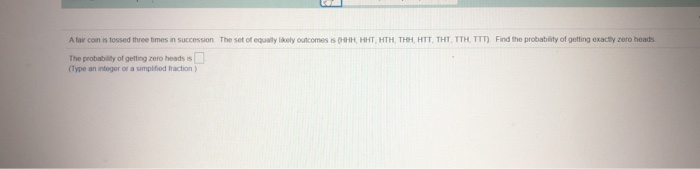A far coin is tossed three times in succession. The set of equally likely outcomes is (HHH, HHT, HTH, THH, HTT, THT, TTH, TTT). Find the probability of getting exactly zero heads The probability of getting zero heads is (Type an integer or a simplified fraction)

• For a fair coin tossed three times, the eight possible simple events are HHH, HHT, HTH, THH, HTT, THT, TTH, TTT

For a fair coin tossed three times, the eight possible simple events are HHH, HHT, HTH, THH, HTT, THT, TTH, TTT. Let X = number of tails. Find the probability distribution for X by filling in the table showing each possible value of X along with the probability that value occurs. k=0,1,2,3 P(X=k)

• A coin is tossed three times. An outcome is represented by a string of the sort...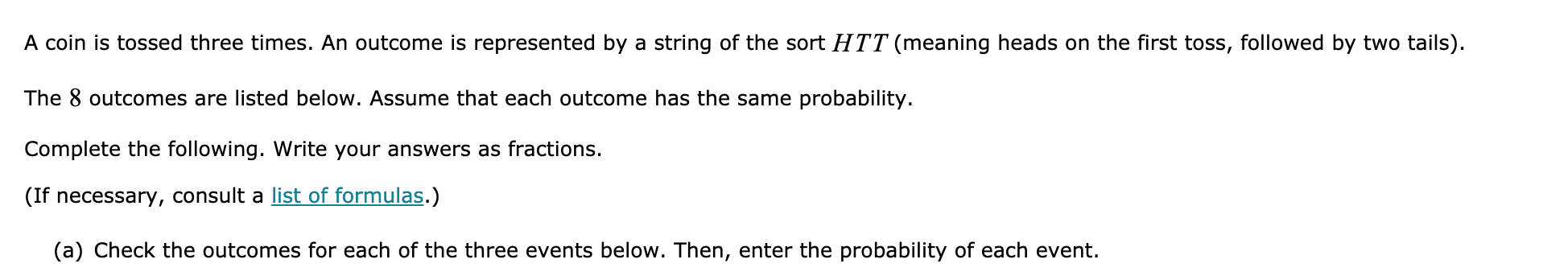A coin is tossed three times. An outcome is represented by a string of the sort HTT (meaning heads on the first toss, followed by two tails). The 8 outcomes are listed below. Assume that each outcome has the same probability. Complete the following. Write your answers as fractions. (If necessary, consult a list of formulas.) (a) Check the outcomes for each of the three events below. Then, enter the probability of each event. (a) Check the outcomes for each...

• Need help: For the experiment described, write the indicated event in set notation

Need help: For the experiment described, write the indicated event in set notation. A coin is tossed three times. Represent the event "the first two tosses come up the same" as a subset of the sample space. A) {(tails, tails), (heads, heads)} B) {hht, tth} C) {hhh, hht, hth, htt, thh, tht, tth, ttt} D) {hhh, hht, tth, ttt}

• Probability Puzzle 3: Flipping Coins If you flip a coin 3 times, the probability of getting...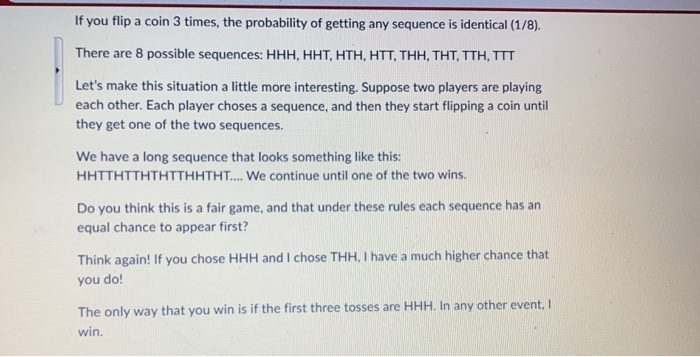Probability Puzzle 3: Flipping Coins If you flip a coin 3 times, the probability of getting any sequence is identical (1/8). There are 8 possible sequences: HHH, HHT, HTH, HTT, THH, THT, TTH, TTT Let's make this situation a little more interesting. Suppose two players are playing each other. Each player choses a sequence, and then they start flipping a coin until they get one of the two sequences. We have a long sequence that looks something like this: HHTTHTTHTHTTHHTHT.......

• Consider the Probability Distribution of the SELECT ALL APPLICABLE CHOICES Number of Heads when Tossing of...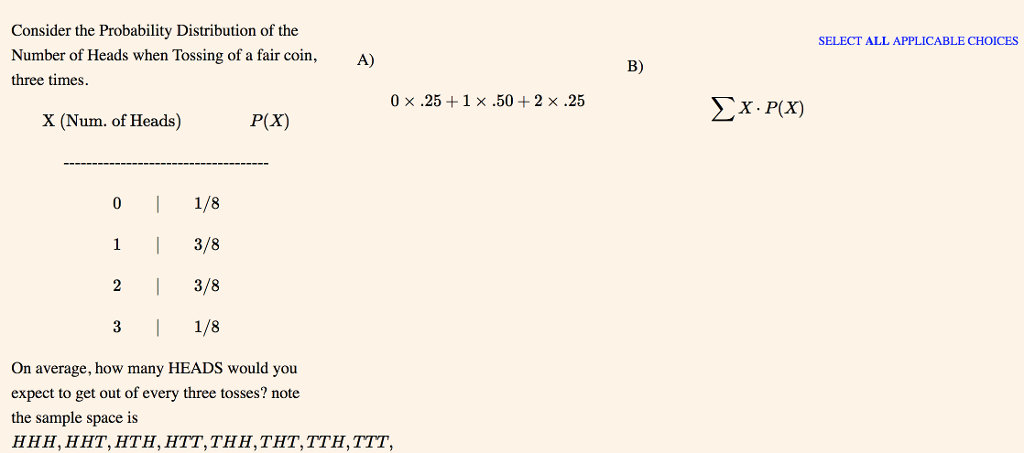Consider the Probability Distribution of the SELECT ALL APPLICABLE CHOICES Number of Heads when Tossing of a fair coin, three times A) B) 0 × .25 + 1 .50 + 2 x .25 X (Num. of Heads) P(X) 0 1/8 1 3/8 2 3/8 3 1/8 On average, how many HEADS would you expect to get out of every three tosses? note the sample space is HHH, HHT, HTH, HTT,THH, THT,TTH, TTT, A person measures the contents of 36 pop...

• A fair coin is tossed 9 times.

A fair coin is tossed 9 times.(A) What is the probability of tossing a tail on the 9th toss, given that the preceding 8 tosses were heads?(B) What is the probability of getting either 9 heads or 9 tails?(A) What is the probability of tossing a tail on the 9th toss, given that the preceding 8 tosses were heads?(B) What is the probability of getting either 9 heads or 9 tails?

• 15. How many possible combination outcomes consist of two heads when you toss a fair coin...

15. How many possible combination outcomes consist of two heads when you toss a fair coin four times? (6.25 points) a. 4 b. 5 c. 6 d. 7 e. None of these

• My No O-5 points LarPCac 92012 Determine whether the sequence is arithmetic. If so, find the common difference d....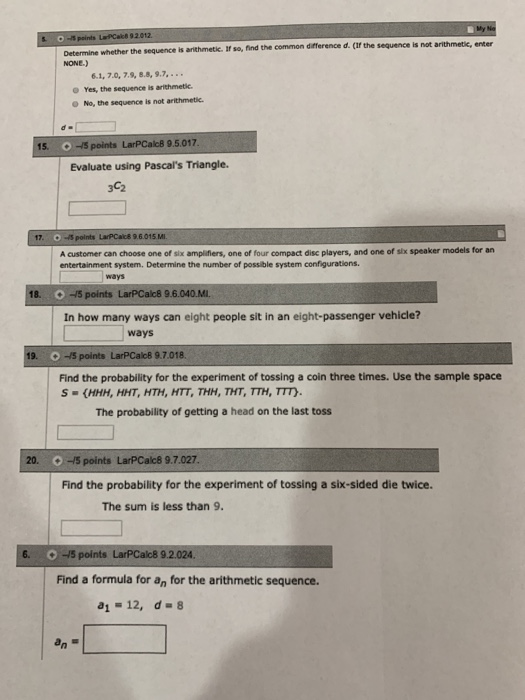My No O-5 points LarPCac 92012 Determine whether the sequence is arithmetic. If so, find the common difference d. (if the sequence is not arithmetic, enter NONE.) 6.1, 7.0, 7.9, 8.8, 9.7, .. Yes, the sequence is arithmetic ONo, the sequence is not arithmetic 45 points LarPCalc8 9.5.017. 15. Evaluate using Pascal's Triangle. 3C2 O-5polnts LarPCalce 9.6.015 M 17 A customer can choose one of six amplifiers, one of four compact disc players, and one of six speaker models for...

• how to calculate d. uty lat thè unit has exactly four rooms, given that it has...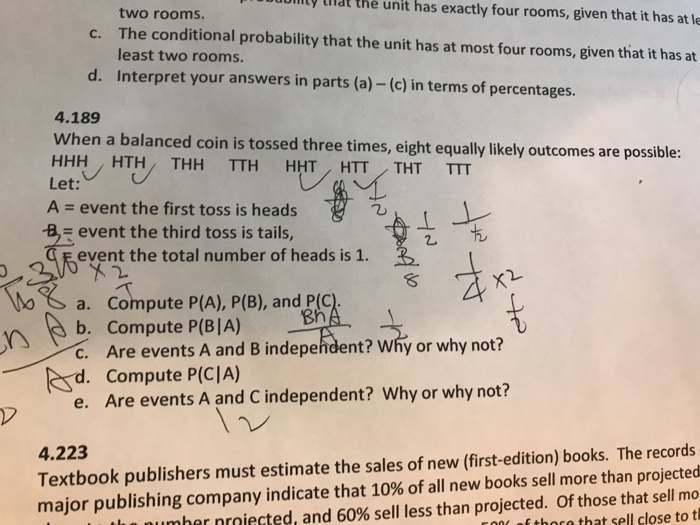how to calculate d. uty lat thè unit has exactly four rooms, given that it has at le two rooms. The conditional probability that the unit has at most four rooms, given that it has at least two rooms. c. d. Interpret your answers in parts (a) - (c) in terms of percentages 4.189 When a balanced coin is tossed three times, eight equally likely outcomes are possible: HHH HTH THH TTH HHT HTT THT TTT Let: A-event the first...